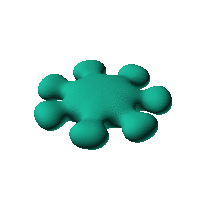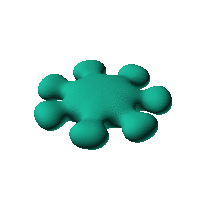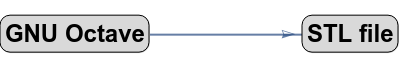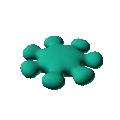Blob Farm# blob18The blob in all its glory:Splat shape, based on inversion on a sphere, https://www.thingiverse.com/thing:3054687Click on the snapshot to download the blob's stl file.Octave Code:
```  function w = f(x2,y2,z2,c,r,e)
x = (x2-c(1))/r(1);
y=(y2-c(2))/r(2);
z=(z2-c(3))/r(3);
k2 = 2.4;
nb = 7; # number of knobs
th = atan2(x,y);
r = sqrt(x.^2+y.^2);
w = ((0.5*cos(nb*th)-0.75).^2 + z.^2 + (r-5).^2).*((x/k2).^2+(y/k2).^2+(z/1.3).^4-1)-3.5 ;
endfunction;

```
GNU Octave